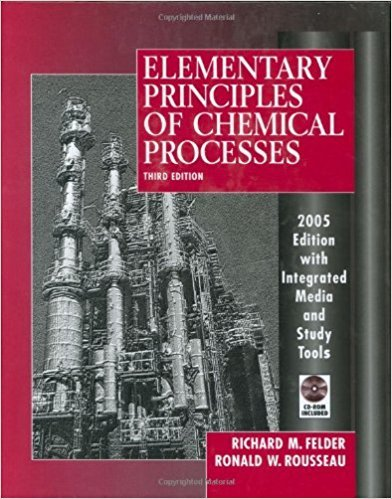×
Get Full Access to Elementary Principles Of Chemical Processes - 3 Edition - Chapter 3 - Problem 3.29
Get Full Access to Elementary Principles Of Chemical Processes - 3 Edition - Chapter 3 - Problem 3.29

×

# A gas stream contains 18.0 mole% hexane and the remainderISBN: 9780471687573 143

## Solution for problem 3.29 Chapter 3

Elementary Principles of Chemical Processes | 3rd Edition

• Textbook Solutions
• 2901 Step-by-step solutions solved by professors and subject experts
• Get 24/7 help from StudySoup virtual teaching assistantsElementary Principles of Chemical Processes | 3rd Edition

4 5 1 277 Reviews
27
1
Problem 3.29

A gas stream contains 18.0 mole% hexane and the remainder nitrogen. The stream flows to a condenser, where its temperature is reduced and some of the hexane is liquefied. The hexane mole Student fraction in the gas stream leaving the condenser is 0.0500. Liquid hexane condensate is recovered at Workbook a rate of 1.50 L/min. Nz Nz C,H"(18.0 mol.%)TC'""(5.00 mol.%) CONDENSER Liquid condensate 1.50 L C6H14 (Iiq)/min (a) What is the flow rate of the gas stream leaving the condenser in mol/min? (Hint: First calculate the molar flow rate of the condensate and note that the rates at which C6H 14 and Nz enter the unit must equal the total rates at which they leave in the two exit streams.) (b) What percentage of the hexane entering the condenser is recovered as a liquid?

Step-by-Step Solution:

Problem 3.29

A gas stream contains 18.0 mole% hexane and the remainder nitrogen. The stream flows to a condenser, where its temperature is reduced and some of the hexane is liquefied. The hexane mole Student fraction in the gas stream leaving the condenser is 0.0500. Liquid hexane condensate is recovered at a rate of 1.50 L/min. (a) What is the flow rate of the gas stream leaving the condenser in mol/min? (Hint: First calculate the molar flow rate of the condensate and note that the rates at which C6H 14 and Nz enter the unit must equal the total rates at which they leave in the two exit streams.) (b) What percentage of the hexane entering the condenser is recovered as a liquid?

Step by step solution

Step 1 of 2

a)

The inlet and outlet conditions of a condenser as follows.From the given,

The density of hexane = 0.659kg/L

Let’s calculate the flow rate of the condensate..

Let’s write the equations for hexane and nitrogen balance.

Hexane balance:Nitrogen balance:Therefore, The gas stream exits the condenser at a rate of 72.3 (mol/min).

____________________________________________________________________________

Step 2 of 2

##### ISBN: 9780471687573

This full solution covers the following key subjects: hexane, condenser, stream, min, condensate. This expansive textbook survival guide covers 13 chapters, and 710 solutions. Since the solution to 3.29 from 3 chapter was answered, more than 1268 students have viewed the full step-by-step answer. This textbook survival guide was created for the textbook: Elementary Principles of Chemical Processes, edition: 3. Elementary Principles of Chemical Processes was written by and is associated to the ISBN: 9780471687573. The answer to “A gas stream contains 18.0 mole% hexane and the remainder nitrogen. The stream flows to a condenser, where its temperature is reduced and some of the hexane is liquefied. The hexane mole Student fraction in the gas stream leaving the condenser is 0.0500. Liquid hexane condensate is recovered at Workbook a rate of 1.50 L/min. Nz Nz C,H"(18.0 mol.%)TC'""(5.00 mol.%) CONDENSER Liquid condensate 1.50 L C6H14 (Iiq)/min (a) What is the flow rate of the gas stream leaving the condenser in mol/min? (Hint: First calculate the molar flow rate of the condensate and note that the rates at which C6H 14 and Nz enter the unit must equal the total rates at which they leave in the two exit streams.) (b) What percentage of the hexane entering the condenser is recovered as a liquid?” is broken down into a number of easy to follow steps, and 134 words. The full step-by-step solution to problem: 3.29 from chapter: 3 was answered by , our top Chemistry solution expert on 11/15/17, 02:42PM.

Unlock Textbook Solution